International
Tables for
Crystallography
Volume F
Crystallography of biological macromolecules
Edited by M. G. Rossmann and E. Arnold

International Tables for Crystallography (2006). Vol. F, ch. 11.5, pp. 238-241   | 1 | 2 |

Section 11.5.7. Experimental results

C. G. van Beek,a R. Bolotovskya§ and M. G. Rossmanna*

aDepartment of Biological Sciences, Purdue University, West Lafayette, IN 47907-1392, USA
Correspondence e-mail:  mgr@indiana.bio.purdue.edu

11.5.7. Experimental results

| top | pdf |

11.5.7.1. Variation of scale factors versus frame number

| top | pdf |

If scale factors are to make physical sense, their behaviour with respect to the frame number has to be in accordance with the known changes in the beam intensity, crystal condition and detector response.

The scaling of a ϕX174 procapsid data set (Dokland et al., 1997) was performed using methods 1and 2as described here and using SCALEPACK (Otwinowski & Minor, 1997) (Fig. 11.5.7.1). Graphs (a) and (b) in Fig. 11.5.7.1have four segments corresponding to four synchrotron beam fills'. All three methods give scale factors within 5% of each other (Figs. 11.5.7.1c and d). However, for the first and last frame of each fill' the results can differ by as much as 15%. Both method 1and SCALEPACK produce physically wrong results in that the scale factors of these frames look like outliers compared to the scale factors of the neighbouring frames. By contrast, method 2provides consistent scale factors for these frames. Although the algorithm used by SCALEPACK for scaling frames with partial reflections has never been disclosed, the similar results obtained by method 1and SCALEPACK suggest that SCALEPACK might be using an algorithm similar to that of method 1(Fig. 11.5.7.1d).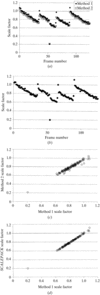Figure 11.5.7.1 | top | pdf |Linear scale factors as a function of frame number for a ϕX174 data set (Dokland et al., 1997). Results from (a) method 1and method 2, (b) SCALEPACK. Comparison of (c) method 2versus method 1, and (d) SCALEPACK versus method 1.

Attempts at scaling a data set of a frozen crystal of HRV14 (Rossmann et al., 1985, 1997) failed with method 1as a result of gaps in the rotation range for the first 20 frames, causing singularity of the normal equations matrix. When frames without useful neighbours were excluded, the cubic symmetry of the crystal was sufficient for successful scaling. In contrast, method 2did not have any problems with the whole data set, and the results obtained with method 2showed greater consistency than those obtained with method 1or SCALEPACK (Fig. 11.5.7.2).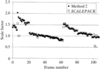Figure 11.5.7.2 | top | pdf |Linear scale factor as a function of frame number for an HRV14 data set (Rossmann et al., 1985, 1997).

The accuracy and robustness of method 2is also demonstrated by the scaling results for a Sindbis virus capsid protein (SCP), residues 114–264 (Choi et al., 1991, 1996). The behaviour of the scale factor with respect to the frame number reflects the anisotropy of the thin plate-shaped crystal (Fig. 11.5.7.3). For the first 40 frames (frame numbers 0 to 39), even-numbered frames have higher scale factors than odd-numbered frames. Data collection was stopped after frame number 39 and restarted. After frame number 39, odd-numbered frames have higher scale factors than even-numbered frames. This effect presumably relates to the use of the two alternating image plates with slightly different sensitivities in the R-axis camera used in the data collection.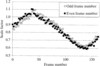Figure 11.5.7.3 | top | pdf |Linear scale factor determined by method 2as a function of frame number for an SCP(114–264) data set (Choi et al., 1991, 1996). The sine-like pattern reflects the anisotropy of a thin plate-shaped crystal.

11.5.7.2. R factor as a function of sum-of-partialities' (method 1)| top | pdf |

In order to determine the limits of tolerance that can be permitted when method 1is used, the R factor was examined as a function of the sum-of-partialities for the ϕX174 procapsid data (Fig. 11.5.7.4). Reflections with sum-of-partialities of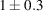were used. The R factor changes sharply when the sum-of-partialities is outside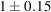. Hence,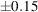were acceptable limits of tolerance for this data set.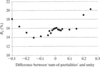Figure 11.5.7.4 | top | pdf |R factor as a function of the difference of calculated sum-of-partialities' and unity for the estimates of full reflections when method 1is used for the scaling and averaging of a ϕX174 data set (Dokland et al., 1997).

11.5.7.3. Statistics for rejecting reflections and data quality as a function of frame number

| top | pdf |

The behaviour of the R factor versus frame number (Fig. 11.5.7.5) is more monotonic when method 1is used compared to method 2. In method 1, the data-quality estimates for neighbouring frames are strongly correlated because the full reflections used in the statistics are obtained by summing partials from consecutive frames. By contrast, in method 2every frame produces estimates of full reflection intensities independently of the neighbouring frames. Therefore, the R factors per frame calculated after scaling with method 2truly represent the data quality for individual frames.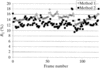Figure 11.5.7.5 | top | pdf |R factor per frame as a function of frame number for a ϕX174 data set (Dokland et al., 1997).

11.5.7.4. Observed versus calculated partiality

| top | pdf |

The relationship between observed and calculated partialities (Fig. 11.5.7.6) deviates from the ideal line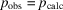, especially for the smaller calculated partialities where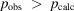. This suggests errors in the measurements of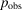or the calculations of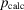. The latter may be improved by a post refinement of the orientation matrix and crystal mosaicity (Rossmann et al., 1979).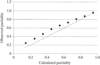Figure 11.5.7.6 | top | pdf |The observed partialities plotted against calculated partialities for a ϕX174 data set (Dokland et al., 1997) processed by method 2. The observed partialities for individual partial reflections were averaged in bins of calculated partialities. The broken line represents the ideal relationship.

11.5.7.5. Anisotropic mosaicity

| top | pdf |

Refinement of the effective mosaicity can show both the anisotropic nature of the crystal (Fig. 11.5.7.7) as well as the impact of radiation damage. The effective mosaicity is the convolution of the mosaic spread of the crystal, the beam divergence and the wavelength divergence of the incident X-ray beam. Hence, X-ray diffraction data collected at a synchrotron-radiation source necessitate the differentiation of the effective mosaicity in the horizontal and vertical planes. A more general approach is the introduction of six parameters reflecting the anisotropic effective mosaicity.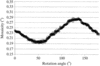Figure 11.5.7.7 | top | pdf |Variation of (unconstrained) mosaicity for a monoclinic crystal of the bacterial virus alpha3 (Bernal et al., 1998) showing the crystal anisotropy.

11.5.7.6. Anomalous dispersion

| top | pdf |

The quality of anomalous-dispersion data can be assessed by calculation of the average scatter, expression (11.5.6.6). The ratios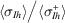and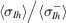should be larger than unity for significant anomalous data (Fig. 11.5.7.8). Note the much larger ratios for the scatter among measurements offor data measured at the absorption edge of Se, as opposed to measurements remote from the edge. The decreasing values of the ratios with resolution are due to the decrease ofvalue, thus causing the error in the measurement ofto approach the difference in intensity of Bijvoet opposites.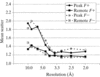Figure 11.5.7.8 | top | pdf |Quality of anomalous-dispersion data for an SeMet derivative of dioxygenase Rieske ferredoxin (Colbert & Bolin, 1999).

References

Choi, H. K., Lee, S., Zhang, Y. P., McKinney, B. R., Wengler, G., Rossmann, M. G. & Kuhn, R. J. (1996). Structural analysis of Sindbis virus capsid mutants involving assembly and catalysis. J. Mol. Biol. 262, 151–167.Google Scholar
Choi, H. K., Tong, L., Minor, W., Dumas, P., Boege, U., Rossmann, M. G. & Wengler, G. (1991). Structure of Sindbis virus core protein reveals a chymotrypsin-like serine proteinase and the organization of the virion. Nature (London), 354, 37–43.Google Scholar
Dokland, T., McKenna, R., Ilag, L. L., Bowman, B. R., Incardona, N. L., Fane, B. A. & Rossmann, M. G. (1997). Structure of a viral procapsid with molecular scaffolding. Nature (London), 389, 308–313.Google Scholar
Otwinowski, Z. & Minor, W. (1997). Processing of X-ray diffraction data collected in oscillation mode. Methods Enzymol. 276, 307–326.Google Scholar
Rossmann, M. G., Arnold, E., Erickson, J. W., Frankenberger, E. A., Griffith, J. P., Hecht, H. J., Johnson, J. E., Kamer, G., Luo, M., Mosser, A. G., Rueckert, R. R., Sherry, B. & Vriend, G. (1985). Structure of a human common cold virus and functional relationship to other picornaviruses. Nature (London), 317, 145–153.Google Scholar
Rossmann, M. G., Leslie, A. G. W., Abdel-Meguid, S. S. & Tsukihara, T. (1979). Processing and post-refinement of oscillation camera data. J. Appl. Cryst. 12, 570–581.Google Scholar
Rossmann, M. G., Momany, C. A., Cheng, B. & Chakravarty, S. (1997). Unpublished results.Google Scholar